Previous: Reversing a Path's Direction  Up: Selecting and Editing Objects  Next: Path Union

# Merging Paths

Multiple shapes can be merged into a single shape using the menu item Transform->Merge Paths. Note that this is not the same as grouping. Moves (gaps) will be placed between the last control point of one path and the first control point of the next path. Any pattern in the selection will be first converted to a full path before merging.

Once the shape has been merged, it can then be edited. If the original shapes had different styles, the new shape will retain the style of the first shape (the lowest one in the stack). For example, in Figure 8.32(a) there are two paths with different styles. Figure 8.32(b) shows the single path created from merging the two original paths. Since the first path used the even-odd winding rule, the new shape has a hole in it.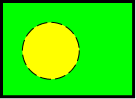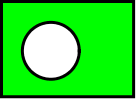(a) (b)
Figure 8.32: Merging two paths: (a) the first path has a solid line pattern, a green fill colour and even-odd winding rule, and the second path has a dashed line pattern and a yellow fill colour; (b) resulting merged path has a solid line pattern, green fill colour and even-odd winding rule.

The same applies if one or more of the selected objects is a text-path. For example, in Figure 8.33(a) there are two text-paths. These are merged to form a single text-path shown in Figure 8.33(b). Note that the text from the second text-path is lost. The resulting path is shown in edit mode in Figure 8.33(c) to illustrate the underlying path. A mixture of paths and text-paths can be merged. The resulting object will be a text-path if the first object to be merged is a text-path, otherwise it will be a path.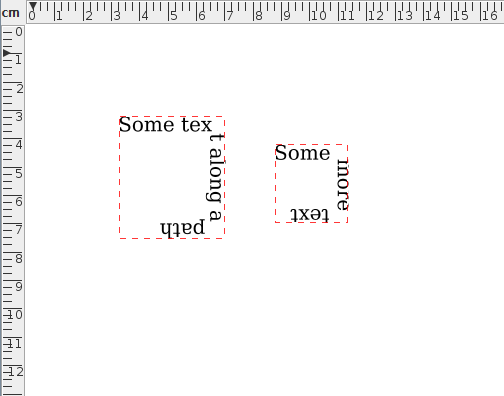(a)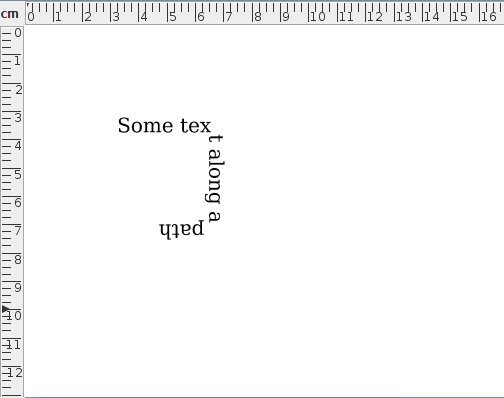(b)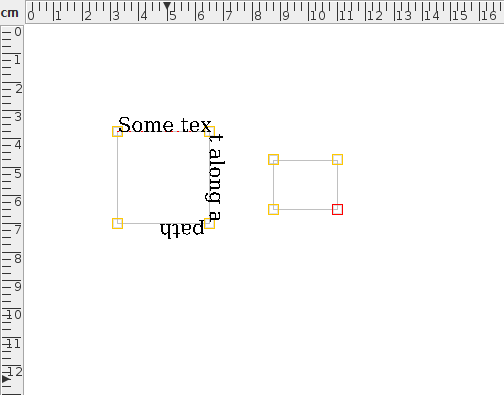(c)
Figure 8.33: Merging two text-paths: (a) the first path is on the left and the second path is on the right; (b) resulting merged path; (c) resulting merged path in edit mode to illustrate the underlying path.

Paths are merged according to their stacking order. For example, in Figure 8.34(a) there are two paths, both with a bar start marker, and an arrow end marker. The path on the right is further back in terms of the stacking order. (That is, it gets painted on the canvas before the other path.) Figure 8.34(b) shows the result of merging the two paths--the left hand path has been appended to the right hand path. Figure 8.34(c) shows the same two paths as in Figure 8.34(a) except that now the left path is the backmost object. There is no visible difference between Figure 8.34(a) and Figure 8.34(c), but the result of merging the paths in Figure 8.34(c) (see Figure 8.34(d)) is different to Figure 8.34(b)--the right hand path has been appended to the left hand path.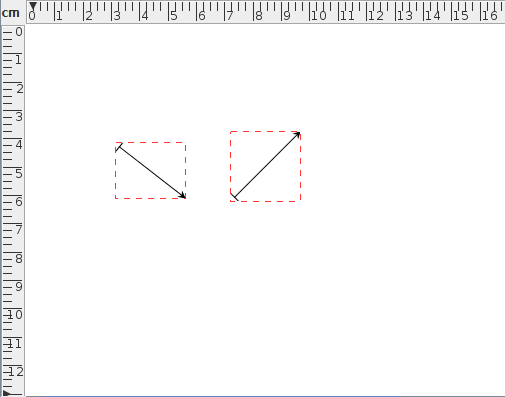(a)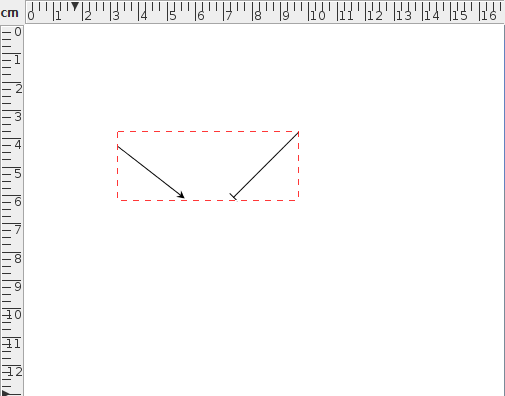(b)(c)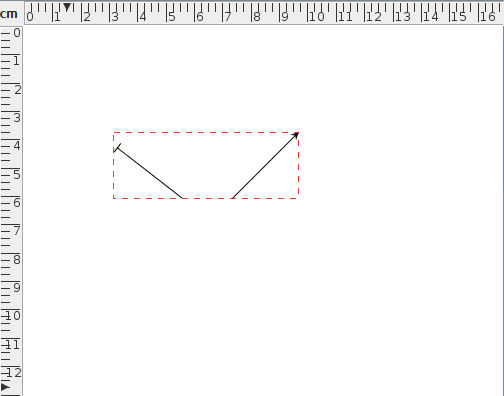(d)
Figure 8.34: Paths are merged according to the stacking order: (a) two straight line paths where the path on the right is at the back of the stack; (b) new single path resulting from merging the two paths in (a); (c) same as (a) but the path on the left is at the back of the stack; (d) new single path resulting from merging the two paths in (c).